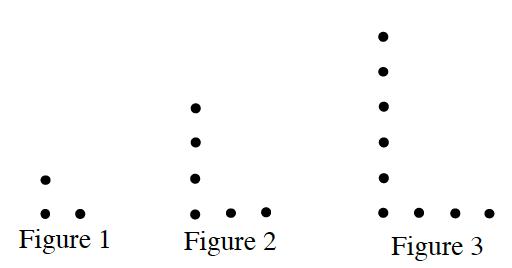### Home > MC1 > Chapter 2 > Lesson 2.1.1 > Problem2-12

2-12.

Copy the dot pattern below on your paper.1. Draw the 4th and 5th figures.

Three dots are added each time. Find where each dot is added.

2. How many dots would be in the 30th figure? Explain how you know.

The thirtieth figure has $90$ dots. Remember to explain how you know.